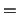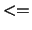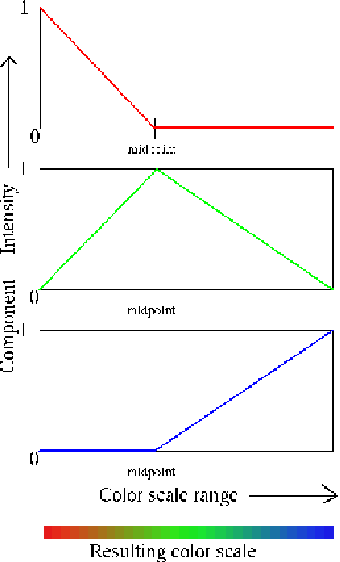Next: Materials Up: Coloring Methods Previous: Coloring by color categories   Contents   Index

## Color scale

Several of the coloring methods, including `Beta', `Charge', and `Occupancy', describe a range of floating point values rather than a set of names. These are colored via the color scale, which is a list of 1024 smoothly changing colors. There are many color gradations available. All of them consist of transformations of three colors. For instance, ``RGB'' colors the smallest value red, values near the middle of the scale are green, and the largest values are blue. Colors in-between are linear mixes of the two colors. The list of available gradations is given below.

Table 5.4: Available Color Scale Gradations.
 Method Description RGB small=red, middle=green, large=blue BGR small=blue, middle=green, large=red RWB small=red, middle=white, large=blue BWR small=blue, middle=white, large=red RWG small=red, middle=white, large=green GWR small=green, middle=white, large=red GWB small=green, middle=white, large=blue BWG small=blue, middle=white, large=green BlkW small=black, large=white WBlk small=white, large=black

The minimum of the range of values is linearly scaled and shifted to start at 0 and end at 1. Assume the color scale is RGB. For a given value of x in the scale range [0..1], the RGB value is found first from a linear scaling based on the midpoint. If x0, R is 1 (for maximum red). This continues linearly until xmidpoint, at which point, R is 0 and stays 0. The green component is 0 at both x0 and x1 and is 1 at the midpoint. Linear scaling occurs in between. The blue component is 0 for xmidpoint, and 1 for x1.An additional term, ``min'', is added to each of the component terms before they are merged. This shifts the final colors more towards white or black. Min can take on values from -1 to 1.There is only one color scale used at a time so it is impossible to display objects colored by multiple different color scales.Next: Materials Up: Coloring Methods Previous: Coloring by color categories   Contents   Index
vmd@ks.uiuc.edu